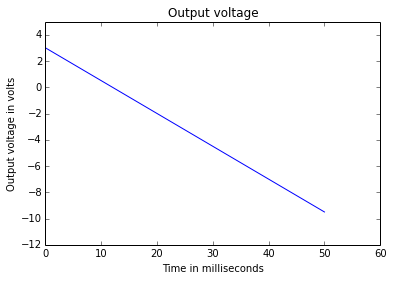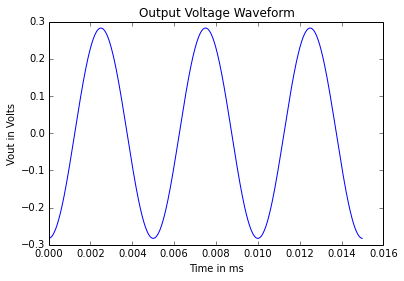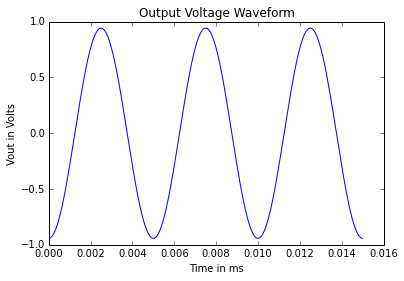# Chapter-5 : General Applications Of Op-Amps¶

## Example 5.1 - Page 156¶

In :
from numpy import pi
import math
# Given data
fo= 15 # in kHz
fo= fo*10**3 # in Hz
C=0.01 # in micro F
C=C*10**-6 # in F
L= 1/(4*pi**2*fo**2*C) # in H
L=math.ceil(L*10**3) # in mH
# Let L be of 12 mH and internal resistance 30 ohm
R=30 # internal resistance in ohm
XL= 2*pi*L*10**-3*fo
Q= XL/R
R_P= Q**2*R # in ohm
# If
R1=100 # in ohm
# Formula L= R_f*R_P/(R1*(R_f+R_P))
R_f= R1*L*R_P/(R_P-R1*L) # in ohm
R_f=R_f*10**3 # in kohm
R_f= 1.2 # in k ohm (Standard value)
print "The values of component chosen are:-"
print "Value of L   = %0.f mH" %L
print "Value of C   = %0.2f micro F" %(C*10**6)
print "Value of R_f = %0.1f k ohm" %R_f
print "Value of R1  = %0.f ohm" %R1

The values of component chosen are:-
Value of L   = 12 mH
Value of C   = 0.01 micro F
Value of R_f = 1.2 k ohm
Value of R1  = 100 ohm


## Example 5.2 - Page 159¶

In :
from __future__ import division
# Given data
Rf= 12 # in k ohm
Rs1= 12 # in k ohm
Rs2= 2 # in k ohm
Rs3= 3 # in k ohm
Vi1= 9 # in volt
Vi2= -3 # in volt
Vi3= -1 # in volt
Vout= -Rf*(Vi1/Rs1+Vi2/Rs2+Vi3/Rs3) # in volt
print "Output voltage = %0.f volt" %Vout

Output voltage = 13 volt


## Example 5.3 - Page NO 159¶

In :
# Given expression Vout= -2*V1+3*V2+4*V3
# For an operational amplifier
# Vout= -Rf*[V1/R1+V2/R2+V3/R3]
# Compare the above expression with the given expression for the output
r_1=2 # value of Rf/R1
r_2=3 # value of Rf/R2
r_3=4 # value of Rf/R3
# Resistance R3 will be minimum value of 10 k ohm
R3=10 # in k ohm
Rf= r_3*R3 # in k ohm
R2= Rf/r_2 # in k ohm
R1= Rf/r_1 # in k ohm
print "Value of Rf = %0.f k ohm" %Rf
print "Value of R2 = %0.2f k ohm" %R2
print "Value of R1 = %0.f k ohm" %R1

Value of Rf = 40 k ohm
Value of R2 = 13.33 k ohm
Value of R1 = 20 k ohm


## Example : 5.4 - Page No 159¶

In :
# Given data
V1= 2 # in volt
V2= -1 # in volt
# Let R1= (R||R)/(R+(R||R))= (R/2)/(R+R/2) = 1/3
R1=1/3
Vs1= V1*R1 # in volt
# Let R2= (1+Rf/R)= (1+2*R/R)= 3
R2= 3
Vo_desh= Vs1*R2 # in volt
Vs2= V2*R1 # in volt
Vo_doubleDesh= Vs2*R2 # in volt
V_out= Vo_desh+Vo_doubleDesh # in volt
print "Output voltage = %0.f volt" %V_out

Output voltage = 1 volt


## Example : 5.5 - Page No 160¶

In :
# Given expression Vout= 10*(V2-V1)
# For a differential amplifier circuit
# Vout= Rf/R*(V2-V1)
# Compare the above expression with the given expression for the output, we have
RfbyR= 10
R=10 # minimum value of resistancce to be used in kohm
Rf= RfbyR * R # in k ohm
print "Value of Rf = %0.f k ohm" %Rf

Value of Rf = 100 k ohm


## Example : 5.7 - Page No 164¶

In :
from __future__ import division
# Given data
R1= 50 # in kohm
# Let us choose
R3= 15 # in k ohm
R4= R3
# For minimum differential voltage gain
R1_max= R1 # since Ad will be minimum only when R1 will be maximum
# Putting values of Ad and R1 in eq(i)
R2= (Ad-1)*R1/2 # in k ohm
# For maximum differential voltage gain
# Putting values of Ad and R2 in eq(i)
R1= 2*R2/(Ad_max-1) # in k ohm
R1=int(R1)
# For maximum value of Ad, R1 will have minimum value , therefore
R1_min= 1 # in kohm
print "Value of R1_min = %0.f k ohm" %R1_min
print "Value of R1     = %0.f-50 k ohm potentiometer" %R1
print "Value of R2     = %0.f k ohm" %R2
print "Value of R3     = %0.f k ohm" %R3
print "Value of R4     = %0.f k ohm" %R4

Value of R1_min = 1 k ohm
Value of R1     = 1-50 k ohm potentiometer
Value of R2     = 100 k ohm
Value of R3     = 15 k ohm
Value of R4     = 15 k ohm


## Example : 5.10 - Page No 179¶

In :
# Given data
Vin= 10 # in volt
R=2.2 # in k ohm
R=R*10**3 #in ohm
T= 1 # in ms
T=T*10**-3 # in second
C=1 # in micro F
C=C*10**-6 # in F
I= Vin/R # in volt
V= I*T/C # in V
print "The output voltage at the end of the pulse = %0.3f volt" %V
print "The closed-loop time constant = %0.f second " %RC_desh

The output voltage at the end of the pulse = 4.545 volt
The closed-loop time constant = 220 second


## Example : 5.11 - Page No 180¶

In :
# Given data
C=0.01 # in micro F
C=C*10**-6 # in F
# Vout/V1= (Rf/R1)/(1+s*C*Rf)
# substituting s= j*omega we have
# Vout/V1 = (Rf/R1)/sqrt((omega*C*Rf)**2+1)
# At omega=0
# Vout/V1= Rf/R1
# Formula omega= 1/(C*Rf)
Rf= 1/(C*omega) # in ohm
Rf= Rf*10**-3 # in k ohm
# 20*log10(Rf/R1) = 20
R1= Rf/10 # in k ohm
print "Value of Rf = %0.f k ohm" %Rf
print "Value of R1 = %0.f k ohm" %R1

Value of Rf = 10 k ohm
Value of R1 = 1 k ohm


## Example : 5.12 Page No 180¶

In :
%matplotlib inline
import matplotlib.pyplot as plt
import numpy as np
#Given Data :
R=40*1000 #in ohm(assumed)
Vout=3 #in Volt
V1=Vout #in Volt
V2=Vout #in Volt
plt.plot([0,50],[3,-9.5])
plt.title('Output voltage')
plt.xlabel('Time in milliseconds')
plt.ylabel('Output voltage in volts')
plt.axis([0, 60, -12, 5])
plt.show()
print "Assuming Ideal op-amp, sketch for Vout is shown in figure."Assuming Ideal op-amp, sketch for Vout is shown in figure.


## Example : 5.13 - Page No 185¶

In :
from sympy import symbols, simplify, sin
t = symbols('t')
# Given data
R= 50*10**3 # in ohm
C= 2*10**-6 # in F
CR= C*R #scale factor
# Vout= -CR*dvc/dt= -CR*d/dt(10*sin(4000*pi*t))
print "Output voltage : 12.56*cos(4000*pi*t) mV"

Output voltage : 12.56*cos(4000*pi*t) mV


## Example : 5.14 - Page No 185¶

In :
from __future__ import division
%matplotlib inline
from sympy import symbols, simplify, sin
import matplotlib.pyplot as plt
import numpy as np
# Given data
fa= 1 # in kHz
fa=fa*10**3 # in Hz
Vp=1.5 # in volt
f= 200 # in Hz
C=0.1 # in micro F
C=C*10**-6 # in F
R= 1/(2*np.pi*fa*C) # in ohm
R=R*10**-3 # in k ohm
R=np.floor(R*10)/10 # in k ohm
fb= 20*fa # in Hz
R_desh= 1/(2*np.pi*fb*C) # in ohm
# Let
R_desh= 82 # in ohm
R_OM= R # in k ohm
print "Value of R_OM = %0.1f k ohm" %R_OM
CR= C*R
# Vin= Vp*sin(omega*t)= 1.5*sin(400*t)
# v_out= -CR*diff(v_in) = -0.2827 Cos(400*pi*t)# in micro volt
print "Output Voltage = -0.2827 Cos(400*pi*t)"
t = np.arange(0, .015, 1.0/44100)
v_out=-0.2827*np.sin(400*np.pi*t+np.pi/2)# in micro volt
plt.plot(t,v_out)
plt.title('Output Voltage Waveform')
plt.xlabel('Time in ms')
plt.ylabel('Vout in Volts')
print "Output Voltage waveform is shown in figure."

Value of R_OM = 1.5 k ohm
Output Voltage = -0.2827 Cos(400*pi*t)
Output Voltage waveform is shown in figure.## Example : 5.15 - Page No 186¶

In :
from __future__ import division
%matplotlib inline
from sympy import symbols, simplify, sin
import matplotlib.pyplot as plt
import numpy as np
from math import sin
t = symbols('t')
# Given data
Vp= 1 # in volts
f= 1000 # in Hz
R= 1.5*10**3 # in ohm
C= 0.1*10**-6 # in F

Output Voltage = -0.942 Cos(400*pi*t)